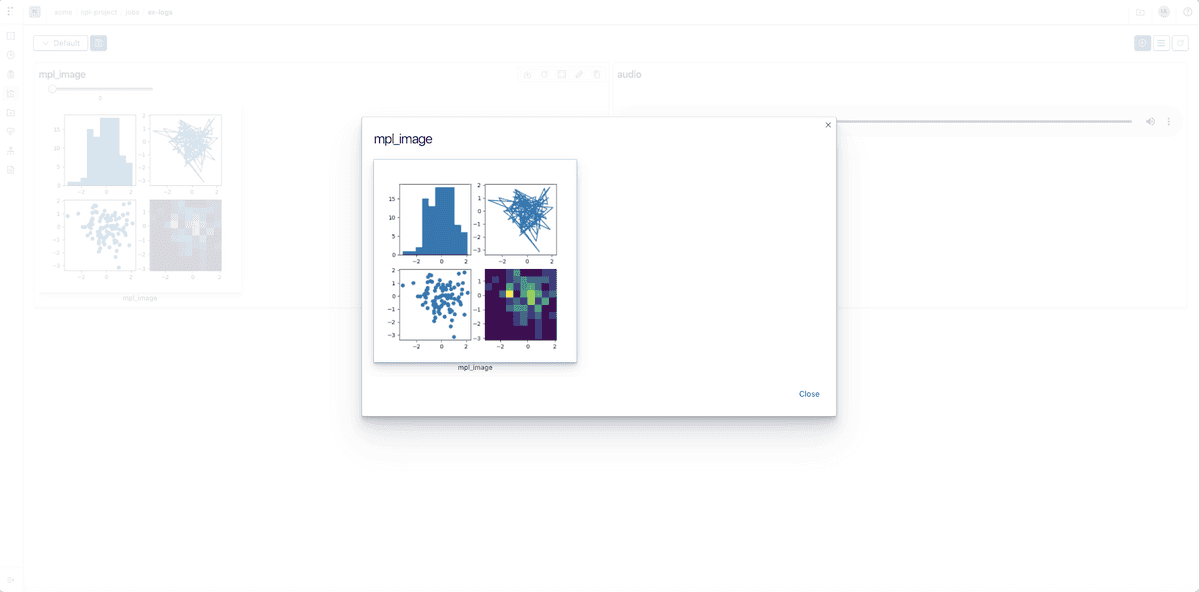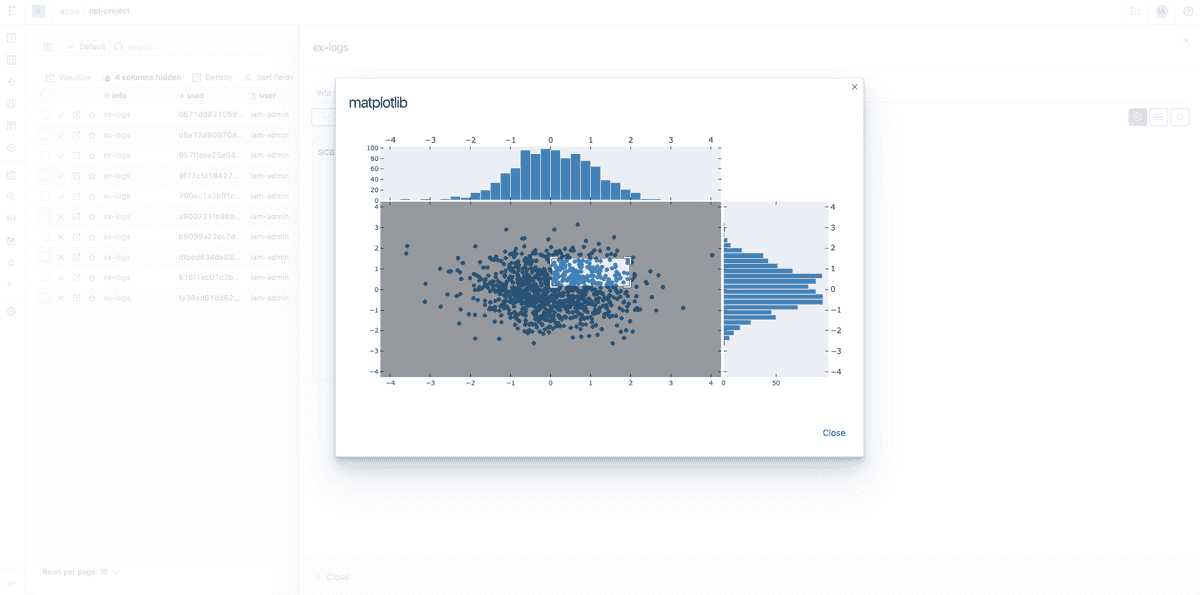You can log and consume figures generated by Matplotlib directly to Polyaxon.

## Tracking

You can log any figure generated by Matplotlib as images images or as Plotly charts.

## Dashboard

Any chart that is logged during the lifetime of your jobs or experiments can be rendered automatically in Polyaxon UI.

## In notebooks

You can also consume any events or charts tracked in your experiments using the Python Library to programmatically visualize results in notebooks.

All charts and events are stored on your artifacts store, and follow any networking or security policy you set for your cluster. You can download any chart tracked to either render it manually or to archive it to a different location using the Python Library

## Example logging Matplotlib figure as an image

### Python Script

``````import matplotlib.pyplot as plt
import numpy as np

from polyaxon import tracking

tracking.init()

np.random.seed(19680801)
data = np.random.randn(2, 100)

figure, axs = plt.subplots(2, 2, figsize=(5, 5))
axs[0, 0].hist(data)
axs[1, 0].scatter(data, data)
axs[0, 1].plot(data, data)
axs[1, 1].hist2d(data, data)

tracking.log_mpl_image(figure, 'mpl_image')``````

### Example as an executable component

``````version: 1.1
kind: component
name: mpl-image
run:
kind: job
init:
- file:
filename: script.py
content: |
import matplotlib.pyplot as plt
import numpy as np
from polyaxon import tracking

tracking.init()
np.random.seed(19680801)
data = np.random.randn(2, 100)

figure, axs = plt.subplots(2, 2, figsize=(5, 5))
axs[0, 0].hist(data)
axs[1, 0].scatter(data, data)
axs[0, 1].plot(data, data)
axs[1, 1].hist2d(data, data)

tracking.log_mpl_image(figure, 'mpl_image')
container:
image: 'polyaxon/polyaxon-examples:ml'
workingDir: '{{ globals.artifacts_path }}'
command: [python3, -u, script.py]``````

### Result

In the `dashboards` tab, create a new image widget## Example logging Matplotlib figure as a Plotly chart

### Python script

``````import matplotlib.pyplot as plt
import numpy as np

from polyaxon import tracking

x = np.random.randn(1000)
y = np.random.randn(1000)

left, width = 0.1, 0.65
bottom, height = 0.1, 0.65
spacing = 0.005

rect_scatter = [left, bottom, width, height]
rect_histx = [left, bottom + height + spacing, width, 0.2]
rect_histy = [left + width + spacing, bottom, 0.2, height]

plt.figure(figsize=(8, 8))

ax_scatter = plt.axes(rect_scatter)
ax_scatter.tick_params(direction='in', top=True, right=True)
ax_histx = plt.axes(rect_histx)
ax_histx.tick_params(direction='in', labelbottom=False)
ax_histy = plt.axes(rect_histy)
ax_histy.tick_params(direction='in', labelleft=False)
ax_scatter.scatter(x, y)

binwidth = 0.25
lim = np.ceil(np.abs([x, y]).max() / binwidth) * binwidth
ax_scatter.set_xlim((-lim, lim))
ax_scatter.set_ylim((-lim, lim))

bins = np.arange(-lim, lim + binwidth, binwidth)
ax_histx.hist(x, bins=bins)
ax_histy.hist(y, bins=bins, orientation='horizontal')

ax_histx.set_xlim(ax_scatter.get_xlim())
ax_histy.set_ylim(ax_scatter.get_ylim())
tracking.log_mpl_plotly_chart(name='scatter', figure=plt)``````

### Example as an executable component

``````version: 1.1
kind: component
name: mpl-to-plotly
run:
kind: job
init:
- file:
filename: script.py
content: |
import matplotlib.pyplot as plt
import numpy as np

from polyaxon import tracking

tracking.init()

x = np.random.randn(1000)
y = np.random.randn(1000)

left, width = 0.1, 0.65
bottom, height = 0.1, 0.65
spacing = 0.005

rect_scatter = [left, bottom, width, height]
rect_histx = [left, bottom + height + spacing, width, 0.2]
rect_histy = [left + width + spacing, bottom, 0.2, height]

plt.figure(figsize=(8, 8))

ax_scatter = plt.axes(rect_scatter)
ax_scatter.tick_params(direction='in', top=True, right=True)
ax_histx = plt.axes(rect_histx)
ax_histx.tick_params(direction='in', labelbottom=False)
ax_histy = plt.axes(rect_histy)
ax_histy.tick_params(direction='in', labelleft=False)
ax_scatter.scatter(x, y)

binwidth = 0.25
lim = np.ceil(np.abs([x, y]).max() / binwidth) * binwidth
ax_scatter.set_xlim((-lim, lim))
ax_scatter.set_ylim((-lim, lim))

bins = np.arange(-lim, lim + binwidth, binwidth)
ax_histx.hist(x, bins=bins)
ax_histy.hist(y, bins=bins, orientation='horizontal')

ax_histx.set_xlim(ax_scatter.get_xlim())
ax_histy.set_ylim(ax_scatter.get_ylim())
tracking.log_mpl_plotly_chart(name='scatter', figure=plt)
container:
image: 'polyaxon/polyaxon-examples:ml'
workingDir: '{{ globals.artifacts_path }}'
command: [python3, -u, script.py]``````

### Result

In the `dashboards` tab, create a new custom chart widget